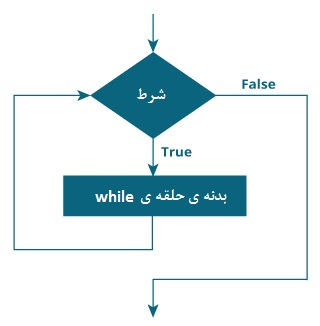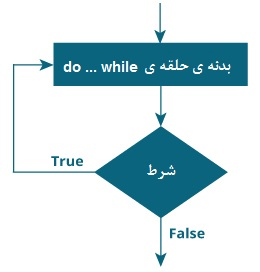# Loops are used in programming to repeat a specific block of code. In this tutorial, you will learn to use the while and do… while loops in Java programming.

Repeat a loop until a certain condition is met (bet is false).

Rings are what make computers interesting machines. Suppose you have to print a sentence 50 times on the screen. You can do this 50 times using the print command (without using a ring). How do you want to print a sentence a million times? So you have to use the rings.

This is just a very simple example. Here you will learn to use the while and do… while loops to write interesting programs.

## While loop in Java

The structure of the while loop is as follows:

while (testExpression) {

// codes inside body of while loop

}

### How does the while loop work?

The condition in parentheses is a boolean expression.

If the condition is true,

• The expressions are executed inside the body of the loop.
• Then, the condition is re-evaluated.

This process continues until the condition is evaluated incorrectly.

If the condition is misjudged,

• The loop ends.

### While loop flowchartExample 1: while loop

1. // Program to print line 10 times
2. class Loop {
3. public static void main (String [] args) {
4. int i = 1;
5. while (i <= 10) {
6. System.out.println (“Line” + i);
7. ++ i;
8. }
9. }
10. }

Output

Line 1

Line 2

Line 3

Line 4

Line 5

Line 6

Line 7

Line 8

Line 9

Line 10

Note: i inside the loop after 10 repetitions will be equal to 11. Then, the condition i <= 10 is considered false and the while loop ends.

Example 2: while loop

1. // Program to find the sum of natural numbers from 1 to 100.
2. class AssignmentOperator {
3. public static void main (String [] args) {
4. int sum = 0, i = 100;
5. while (i! = 0) {
6. sum + = i; // sum = sum + i;
7. –I;
8. }
9. System.out.println (“Sum =” + sum);
10. }
11. }

Output

Sum = 5050

Here, the value of the sum variable is 0 and the initial value of i is 100. In each iteration of the loop, the value of the variable is summed with i (sum + i), and the value of i decreases by one until i equals 0. For better visualization,

1st iteration: sum = 0 + 100 = 100, i = 99

2nd iteration: sum = 100 + 99 = 199, i = 98

3rd iteration: sum = 199 + 98 = 297, i = 97

… ..…

… ..…

99th iteration: sum = 5047 + 2 = 5049, i = 1

100th iteration: sum = 5049 + 1 = 5050, i = 0

## Do… while loop in Java

The do… while loop is similar to the while loop with one key difference. The body of the do… while loop is executed once before the condition is checked.

Do… while loop structure:

do {

// codes inside body of do while loop

} while (testExpression);

### How does the do… while loop work?

The body of the do… while loop is executed once (before checking the condition). In the next series, the condition is checked.

If the condition is evaluated correctly, the code inside the body of the loop is executed, and the condition is evaluated again. This process continues until the condition is considered incorrect.

When the condition is false, the do… while loop ends.

### Do… while loop flowchartExample 3: do… while loop

The following program calculates the number of numbers that the user enters until login 0.

We used the Scanner object to get input from the user.

1. import java.util.Scanner;
2. class Sum {
3. public static void main (String [] args) {
4. Double number, sum = 0.0;
5. Scanner input = new Scanner (System.in);
6. do {
7. System.out.print (“Enter a number:”);
8. number = input.nextDouble ();
9. sum + = number;
10. } while (number! = 0.0);
11. System.out.println (“Sum =” + sum);
12. }
13. }

Output

Enter a number: 2.5

Enter a number: 23.3

Enter a number: -4.2

Enter a number: 3.4

Enter a number: 0

Sum = 25.0

## Infinite while loop

If the condition is never wrong, the loop body is done indefinitely. Currently the while loop is executed many times (at least in theory). For example

1. while (true) {
2. // body of while loop
3. }

Another example:

1. int i = 100;
2. while (i == 100) {
3. System.out.print (“Hey!”);
4. }